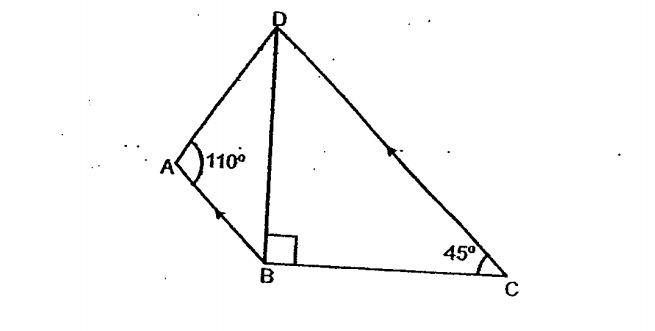# Question 1 of 25

In the figure below, not drawn to scale, ABCD is a trapezium. Find $\angle$ADB.A
10$^\circ$
B
15$^\circ$
C
20$^\circ$
D
25$^\circ$
E
None of the above